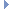OpenMM
Integrator Class Reference

An Integrator defines a method for simulating a System by integrating the equations of motion. More...Inheritance diagram for Integrator:

List of all members.

## Public Member Functions

def __init__
def getStepSize
getStepSize(self) -> double
def setStepSize
Set the size of each time step, in picoseconds.
def getConstraintTolerance
getConstraintTolerance(self) -> double
def setConstraintTolerance
Set the distance tolerance within which constraints are maintained, as a fraction of the constrained distance.
def step
Advance a simulation through time by taking a series of time steps.
def __getstate__
def __setstate__
def __deepcopy__
def __copy__
__copy__(self) -> Integrator

this

## Detailed Description

An Integrator defines a method for simulating a System by integrating the equations of motion.

This is an abstract class. Subclasses define particular integration methods.

Each Integrator object is bound to a particular Context which it integrates. This connection is specified by passing the Integrator as an argument to the constructor of the Context.

## Constructor & Destructor Documentation

 def __init__ ( self, args, kwargs )

## Member Function Documentation

 def __copy__ ( self )

__copy__(self) -> Integrator

 def __deepcopy__ ( self, memo )
 def __getstate__ ( self )
 def __setstate__ ( self, serializationString )
 def getConstraintTolerance ( self )

getConstraintTolerance(self) -> double

Get the distance tolerance within which constraints are maintained, as a fraction of the constrained distance.

Reimplemented in CompoundIntegrator.

 def getStepSize ( self )

getStepSize(self) -> double

Get the size of each time step, in picoseconds. If this integrator uses variable time steps, the size of the most recent step is returned.

Returns:
(double) the step size, measured in ps

Reimplemented in CompoundIntegrator.

 def setConstraintTolerance ( self, tol )

Set the distance tolerance within which constraints are maintained, as a fraction of the constrained distance.

Reimplemented in CompoundIntegrator.

 def setStepSize ( self, size )

Set the size of each time step, in picoseconds.

If this integrator uses variable time steps, the effect of calling this method is undefined, and it may simply be ignored.

Parameters:
 size (double) the step size, measured in ps

Reimplemented in CompoundIntegrator.

 def step ( self, steps )

Advance a simulation through time by taking a series of time steps.

Parameters:
 steps (int) the number of time steps to take

## Member Data Documentation

The documentation for this class was generated from the following file:
• openmm.py